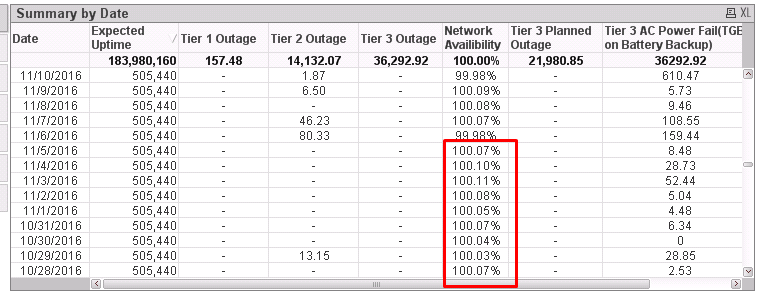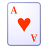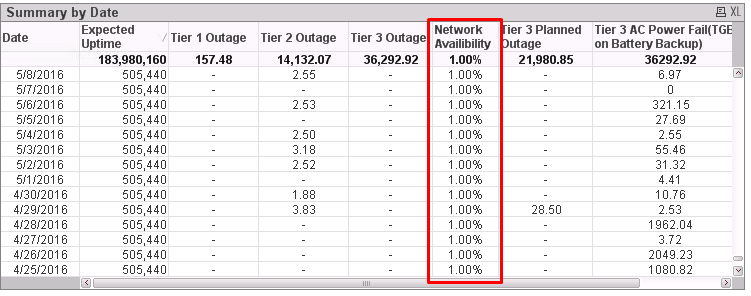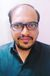# QlikView App Dev

Discussion Board for collaboration related to QlikView App Development.

Announcements
cancel
Showing results for
Did you mean:Not applicable

## How to Round off the percentage values?

Hi All,

I need the below percentage values to get Roundup as 100%.I have Tried by using Round, Floor and Ceil function .it works for 100% but 99.98 % is also turning as 100%

Requirements I need:

100.02: as 100 %

99.92: as either 99.92 or 99%can anyone help me fixing it? so that it will be help full for my learning process

Thanks.

Muthu

1 Solution

Accepted SolutionsPartner

I dont understand the rounding rules you want to apply. 99.98 does not round to 99, it will round to 100, and what does "either 99.98 or 99%"  mean? You need one or the other.

In general,

round(x, y) will round x up or down to the nearest multiple of y. If y is omitted, use 1

ceil(x, y) will round x up to the nearest multiple of y. Likewise y defailts to 1 if omitted.

floor(x, y) will round down to the nearest multiple of y.

To produce 99.98, then use something like

=Round(99.977, 0.01)

To "round" 99.98 to 99, then use

=Floor(99.98, 1)

Similarly for 100.01

=Round(100.01, 1)

=Floor(100.01, 1)

Logic will get you from a to b. Imagination will take you everywhere. - A Einstein
13 RepliesSpecialistNot applicable
Author

But the above Expression is Not working

for your Exapression iam getting the Output asBut i need an out put like

100.01 % as 100%

99.98 % as either 99.98 or 99%

The Expression that I am using:

=Num((1-((Sum([Transceiver Daily Outage Duration])-Sum([Planned Downtime]))

-

(Sum([CPE Daily Outage Duration])+Sum([Tier1 Daily Outage Duration])))

/

Sum([Transceiver Daily Expected Uptime])),'#,##0.00%')Creator III

Hi ,

Change the number format in Number tab of that expression.

Select Fixed and set 0 Decimal. if you are not multiplying the factor with 100 in you expression check

"Show in Percentage in the Number Tab"Not applicable
Author

I have checked your Logic. It is also not working

I am getting the output as 100%  for all fields

even 99.98 % also getting 100%

But I need an output like

100.01 % as 100%

99.98 % as either 99.98 or 99%

Thanks,

MuthuCreator III

Try ceil()  or Floor()Partner

I dont understand the rounding rules you want to apply. 99.98 does not round to 99, it will round to 100, and what does "either 99.98 or 99%"  mean? You need one or the other.

In general,

round(x, y) will round x up or down to the nearest multiple of y. If y is omitted, use 1

ceil(x, y) will round x up to the nearest multiple of y. Likewise y defailts to 1 if omitted.

floor(x, y) will round down to the nearest multiple of y.

To produce 99.98, then use something like

=Round(99.977, 0.01)

To "round" 99.98 to 99, then use

=Floor(99.98, 1)

Similarly for 100.01

=Round(100.01, 1)

=Floor(100.01, 1)

Logic will get you from a to b. Imagination will take you everywhere. - A EinsteinSpecialist

Use thisNum((1-((Sum([Transceiver Daily Outage Duration])-Sum([Planned Downtime]))

-

(Sum([CPE Daily Outage Duration])+Sum([Tier1 Daily Outage Duration])))

/

Sum([Transceiver Daily Expected Uptime])),'#,##0.#0%')Creator II

Hi Muthu,

Try this Exppression,"Round(99.98,0.04)" if it works.

Thank's & Regards,Not applicable
Author